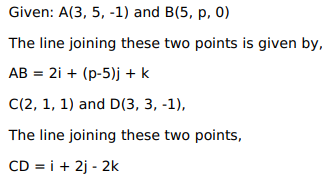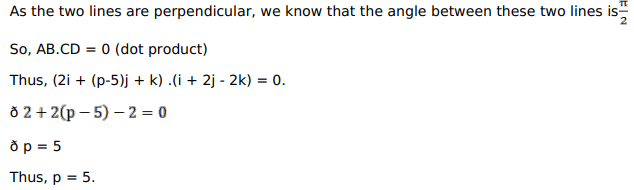# Solve this following

Question:

Find the value of $p$ for which the line through the points $A(3,5,-1)$ and $B(5, p, 0) 9$ is perpendicular to the line through the points $C(2,1,1)$ and $D(3,3,-1)$.

Solution: